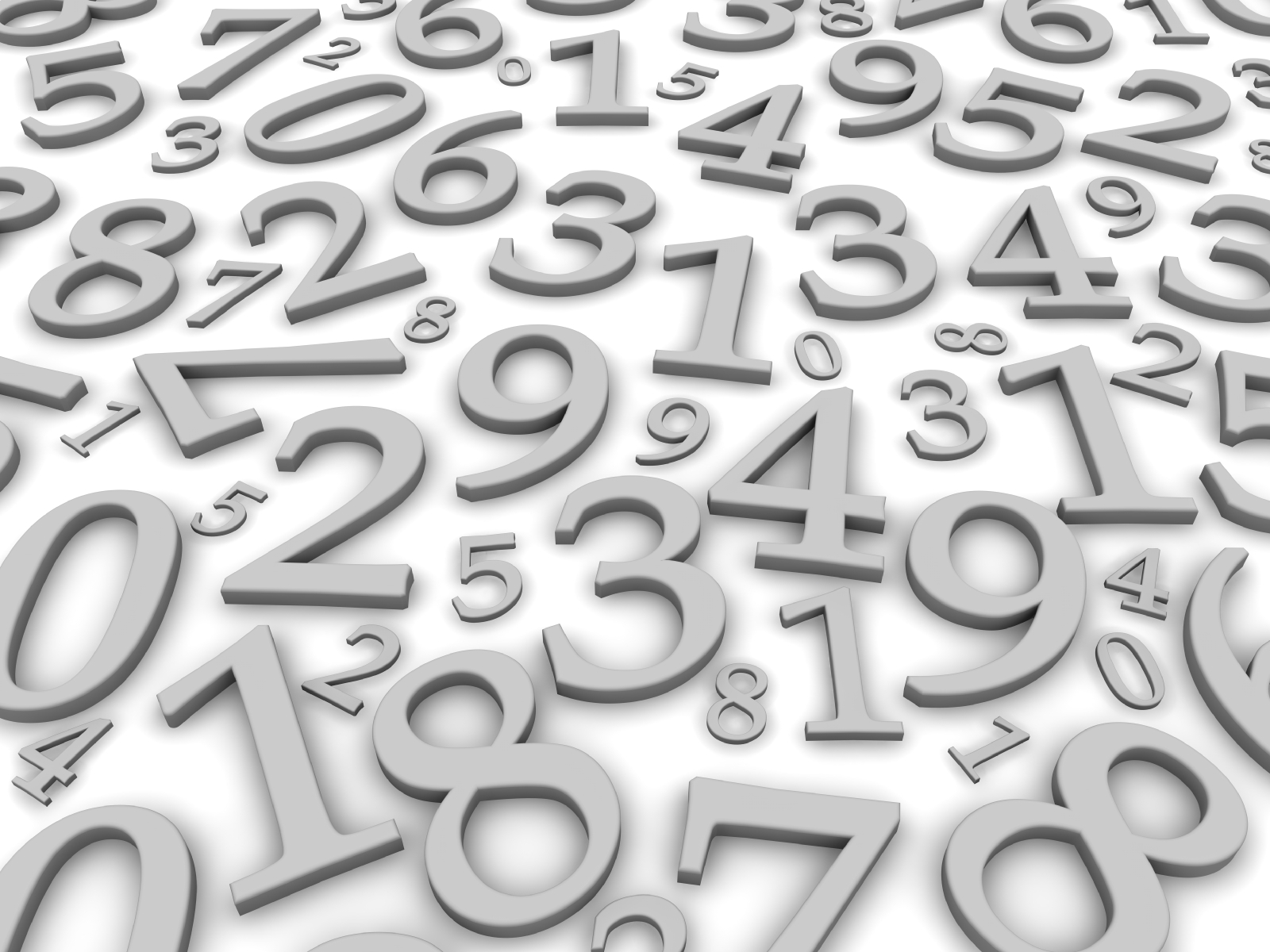# 3- digit ManiaThere are four 3-digit natural numbers, each of them equals the sum of the cubes of its digits.

Three of them are:

153=1+125+27

370=27+343+0

407=64+0+343

Do you know what the fourth one is? It does not begin with 0, otherwise it isn't a 3-digit number.

Hint --- Its not as difficult as it appears. Look at the 3 numbers given.

×